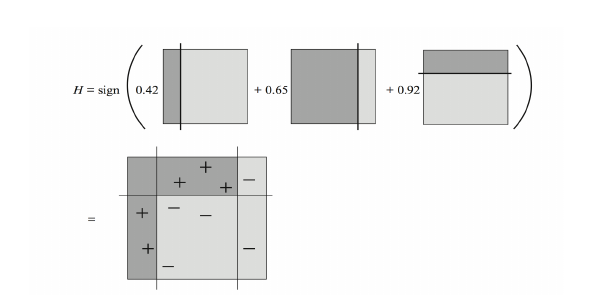# 集成学习之Boosting —— AdaBoost原理

## AdaBoost原理概述

AdaBoost是一个具有里程碑意义的算法，因为其是第一个具有适应性的算法，即能适应弱学习器各自的训练误差率，这也是其名称的由来（Ada为Adaptive的简写）。

AdaBoost的具体流程为先对每个样本赋予相同的初始权重，每一轮学习器训练过后都会根据其表现对每个样本的权重进行调整，增加分错样本的权重，这样先前做错的样本在后续就能得到更多关注，按这样的过程重复训练出M个学习器，最后进行加权组合，如下图所示。

#1. 每轮训练过后如何调整样本权重$w$
2. 如何确定最后各学习器的权重 $\alpha$

## 加法模型 (Additive Model) 和指数损失函数 (Exponential Loss)

$f(x) = \sum_{m=1}^{M}\alpha_{m}G_{m}(x)$

$\min\limits_{(\alpha_{m}\,,\,G_{m})}\sum\limits_{i=1}^{N}L(y_{i}, \,\sum\limits_{m=1}^{M}\alpha_{m}G_{m}(x_{i})) \qquad (1.1)$

AdaBoost采用的损失函数为指数损失，形式如下：
$L(y,\,f(x)) = e^{-yf(x)} \qquad (1.2)$

$(\alpha_{m},G_{m}(x)) = \mathop{\arg\min}\limits_{(\alpha,G)}\sum\limits_{i=1}^{N}e^{-y_{i}f_{m}(x_{i})} = \mathop{\arg\min}\limits_{(\alpha,G)}\sum\limits_{i=1}^{N}e^{-y_{i}(f_{m-1}(x_{i}) + \alpha G(x_{i}))} \qquad (1.3)$

$w_{i}^{(m)} = e^{-y_{i}f_{m-1}(x_{i})}$，由于$w_i^{(m)}$不依赖于$\alpha$$G(x)$，所以可认为其是第m步训练之前赋予每个样本的权重。然而$w_i^{(m)}$依赖于$f_{m-1}(x_i)$，所以每一轮迭代会改变。

$\sum\limits_{i=1}^{N}w_{i}^{(m)}e^{-y_i\alpha G(x_i)} = e^{-\alpha}\sum\limits_{y_{i}=G(x_{i})}w_{i}^{(m)} + e^{\alpha}\sum\limits_{y_i \neq G(x_i)}w_i^{(m)} \qquad\qquad (1.4)$

$= (e^{\alpha} - e^{-\alpha})\sum\limits_{i=1}^Nw_i^{(m)}\mathbb{I}(y_i \neq G(x_i)) + e^{-\alpha}\sum\limits_{i=1}^Nw_i^{(m)} \qquad\qquad\quad (1.5)$

(1) 基学习器$G_m(x)$ :

(2) 下一轮样本权值$w_i^{(m+1)}$

$w_{i}^{(m+1)} = e^{-y_{i}f_{m}(x_{i})} = e^{-y_{i}(f_{m-1}(x_{i}) + \alpha G(x_{i}))} = e^{-y_if_{m-1}(x_i)}e^{-y_i\alpha_mG_m(x_i)} = w_i^{(m)}e^{-y_i\alpha_mG_m(x_i)}$

(3) 各基学习器的系数 $\alpha_m$

$G_m(x)$在训练集上的带权误差率为 $\epsilon_m = \frac{\sum\limits_{i=1}^Nw_i^{(m)}\mathbb{I}(y_i \neq G_m(x_i))}{\sum\limits_{i=1}^Nw_i^{(m)}}$

## AdaBoost算法

1. 初始化权值分布： $w_i^{(1)} = \frac{1}{N}\:, \;\;\;\; i=1,2,3, \cdots N$
2. for m=1 to M:
(a) 使用带有权值分布的训练集学习得到基学习器$G_m(x)$:
$G_m(x) = \mathop{\arg\min}\limits_{G(x)}\sum\limits_{i=1}^Nw_i^{(m)}\mathbb{I}(y_i \neq G(x_i))$
(b) 计算$G_m(x)$在训练集上的误差率：
$\epsilon_m = \frac{\sum\limits_{i=1}^Nw_i^{(m)}\mathbb{I}(y_i \neq G_m(x_i))}{\sum\limits_{i=1}^Nw_i^{(m)}}$
(c) 计算$G_m(x)$的系数： $\alpha_m = \frac{1}{2}ln\frac{1-\epsilon_m}{\epsilon_m}$
(d) 更新样本权重分布： $w_{i}^{(m+1)} = \frac{w_i^{(m)}e^{-y_i\alpha_mG_m(x_i)}}{Z^{(m)}}\; ,\qquad i=1,2,3\cdots N$
其中$Z^{(m)}$是规范化因子，$Z^{(m)} = \sum\limits_{i=1}^Nw^{(m)}_ie^{-y_i\alpha_mG_m(x_i)}$，以确保所有的$w_i^{(m+1)}$构成一个分布。
3. 输出最终模型： $G(x) = sign\left[\sum\limits_{m=1}^M\alpha_mG_m(x) \right]$

## AdaBoost采用指数损失的原因

$E(e^{-yf(x)}|x) = P(y=1|x)e^{-f(x)} + P(y=-1|x)e^{f(x)}$

$\frac{\partial E(e^{-yf(x)}|x)}{\partial f(x)} = -P(y=1|x) e^{-f(x)} + P(y=-1|x)e^{f(x)} = 0\quad ，$
$f(x) = \frac12 log\frac{P(y=1|x)}{P(y=-1|x)}，或写为 P(y=1|x) = \frac{1}{1+e^{-2f(x)}}$

\begin{equation} \begin{aligned} sign(f(x)) &= sign(\frac12 log\frac{P(y=1|x)}{P(y=-1|x)}) \\ & =\left\{\begin{matrix} 1 & \;\; P(y=1|x) > p(y=-1|x)\\ -1 & \;\; P(y=1|x) < P(y=-1|x) \end{matrix}\right. \\&=\mathop{\arg\max} \limits_{y \in\{-1,1\}}P(y|x) \end{aligned} \end{equation}

## Real AdaBoost

\begin{equation} \begin{aligned} E(e^{-yf_m(x)}|x) &= E(e^{-y(f_{m-1}(x) + G(x))}|x) \\ &=E(w \cdot e^{-yG(x)}|x) \\&=e^{-G(x)}P_w(y=1|x) + e^{G(x)}P_w(y=-1|x)\end{aligned} \end{equation}

$G(x)$求导： $G(x) = \frac12log\frac{P_w(y=1|x)}{P_w(y=-1|x)}$

1. 初始化权重分布：$w_i^{(1)} = \frac1N, \qquad i = 1,2 \cdots N$
2. for m=1 to M:
(a) 使用带权重分布的训练集训练基学习器，得到类别概率$P_m(x) = P_w(y=1|x) \;\in [0,1]$
(b) $G_m(x) = \frac12 log\frac{P_m(x)}{1-P_m(x)} \;\in R$
(c) 更新权重分布： $w_i^{(m+1)} = \frac{w_i^{(m)}e^{-y_iG_m(x_i)}}{Z^{(m)}}$
3. 输出最终模型：$G(x) = sign\left[\sum\limits_{m=1}^MG_m(x)\right]$

## 正则化 (Regularization) 和其他

### 1、Shrinkage

$f_m(x) = f_{m-1}(x) + \alpha_m G_m(x)$

$f_m(x) = f_{m-1}(x) + \nu \cdot \alpha_m G_m(x)$

### 3、Weight Trimming

weight trimming不是正则化的方法，其主要目的是提高训练速度。在AdaBoost的每一轮基学习器训练过程中，只有小部分样本的权重较大，因而能产生较大的影响，而其他大部分权重小的样本则对训练影响甚微。Weight trimming的思想是每一轮迭代中删除那些低权重的样本，只用高权重样本进行训练。具体是设定一个阈值 (比如90%或99%)，再将所有样本按权重排序，计算权重的累积和，累积和大于阈值的权重 (样本) 被舍弃，不会用于训练。注意每一轮训练完成后所有样本的权重依然会被重新计算，这意味着之前被舍弃的样本在之后的迭代中如果权重增加，可能会重新用于训练。

## 终末

AdaBoost的原始论文 [Freund & Schapire, 1997] 并非使用了上文中的推导方法，而是基于PAC学习框架下进行解释的。上文的将AdaBoost视为 “加法模型 + 指数损失” 的观点，由斯坦福的几位统计系大牛 [Friedman et al., 2000] 提出，因而这一派也被称为“统计视角”。沿着这个路子，若将指数损失替换为其他损失函数并依此不断训练新学习器，则诞生了Gradient Boosting算法，是为下一篇的主题。

## Reference :

1. Friedman, J., Hastie, T. and Tibshirani, R. Additive logistic regression: a statistical view of boosting
2. Friedman, J., Hastie, T. and Tibshirani, R. The Elements of Staistical Learning
3. 周志华. 《机器学习》
4. 李航. 《统计学习方法》
5. Schapire R. E. Expalining AdaBoost

/

posted @ 2018-05-20 12:36 massquantity 阅读(...) 评论(...) 编辑 收藏